# Unit Circle

 1 What is a Unit Circle? 2 Formula for Unit Circle 3 Calculating Trigonometric Functions using Unit Circle 4 Tips and Tricks 5 Unit Circle Calculator 6 Solved Examples of Unit Circle 7 Important Notes on Unit Circle 8 Practice Questions of Unit Circle 9 Challenging Questions on Unit Circle 10 Maths Olympiad Sample Papers 11 Frequently Asked Questions (FAQs)

We at Cuemath believe that Math is a life skill. Our Math Experts focus on the “Why” behind the “What.” Students can explore from a huge range of interactive worksheets, visuals, simulations, practice tests, and more to understand a concept in depth.

Book a FREE trial class today! and experience Cuemath’s LIVE Online Class with your child.

## What is a Unit Circle?

A unit circle means a circle with radius 1 unit.

A unit circle in math is an important topic which helps us understand the trigonometric functions in the cartesian coordinate plane.

Let us see how to use the Pythagoras theorem and the unit circle to understand the trigonometric functions of sine, cosine and tangent.

## Formula for Unit Circle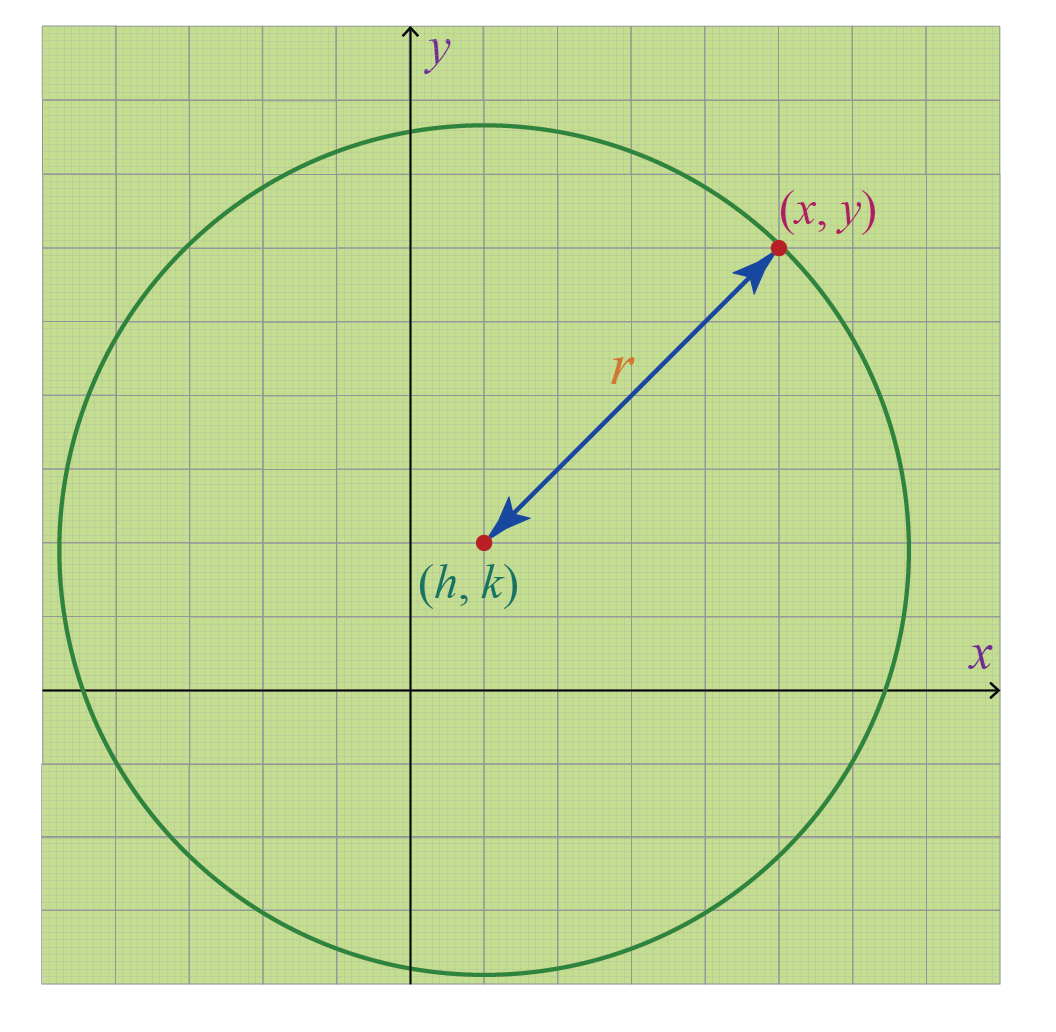The equation of a circle is given by the formula:

 $$(x-h)^2 +(y-k)^2 = r^2$$

where $$(h,k)$$ are the coordinates of the centre in the cartesian plane and r is the radius of the circle.
($$x,y$$) are the points on the circumference of the circle which are at a distance $$r$$ (radius) from the center($$h,k$$).

In case of a unit circle, the centre lies at (0,0) and the radius is 1 unit.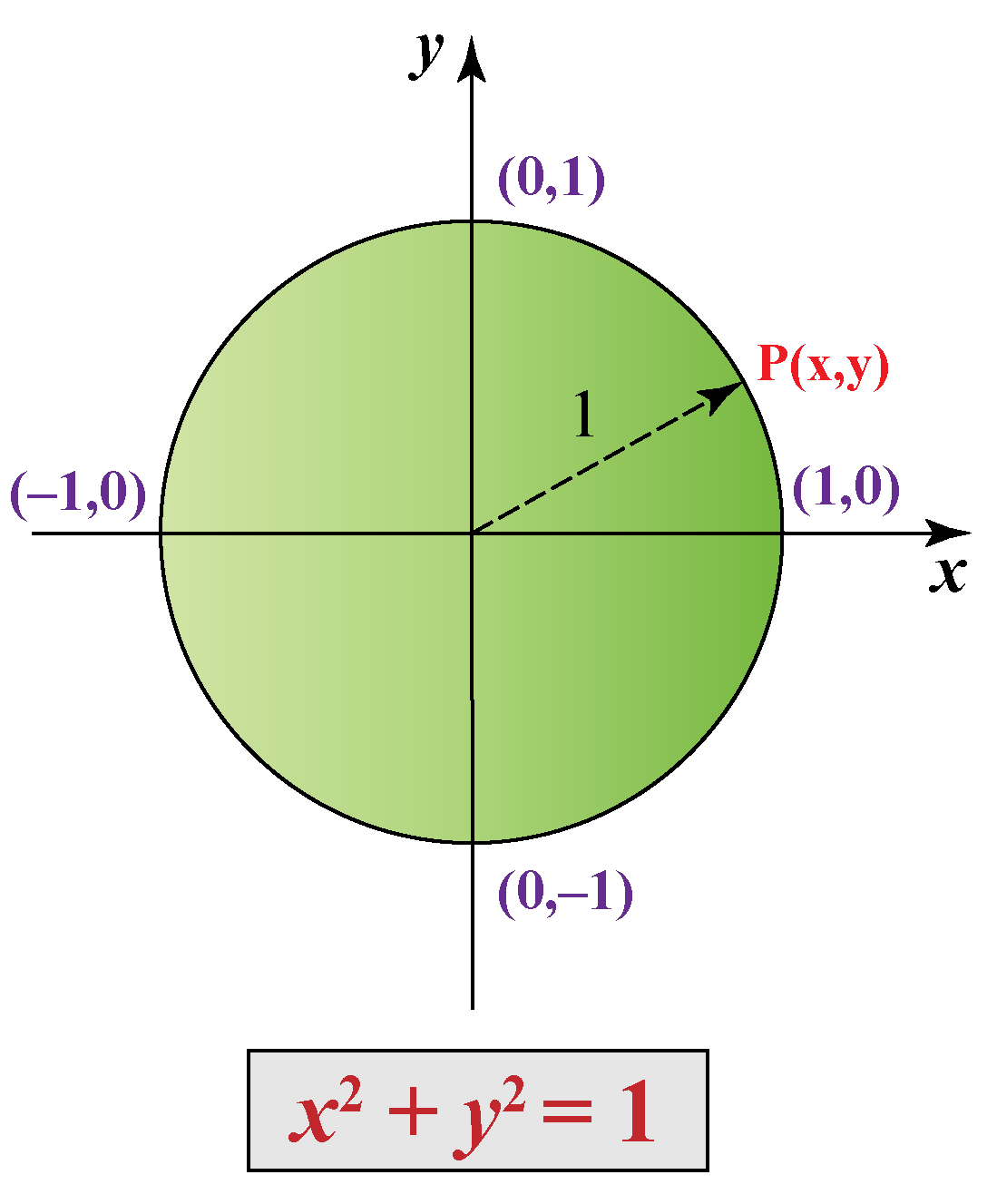Thus, the formula for the unit circle is:

 $$x^2 +y^2 = 1$$

## Calculating Trigonometric Functions Using Unit Circle

We calculate the trigonometric functions sine, cosine and tangent using unit circle.

Let us apply the Pythagoras theorem in a unit circle to understand the trigonometric functions.

Consider a right triangle placed in a unit circle in the cartesian coordinate plane.

The trigonometric functions are shown below.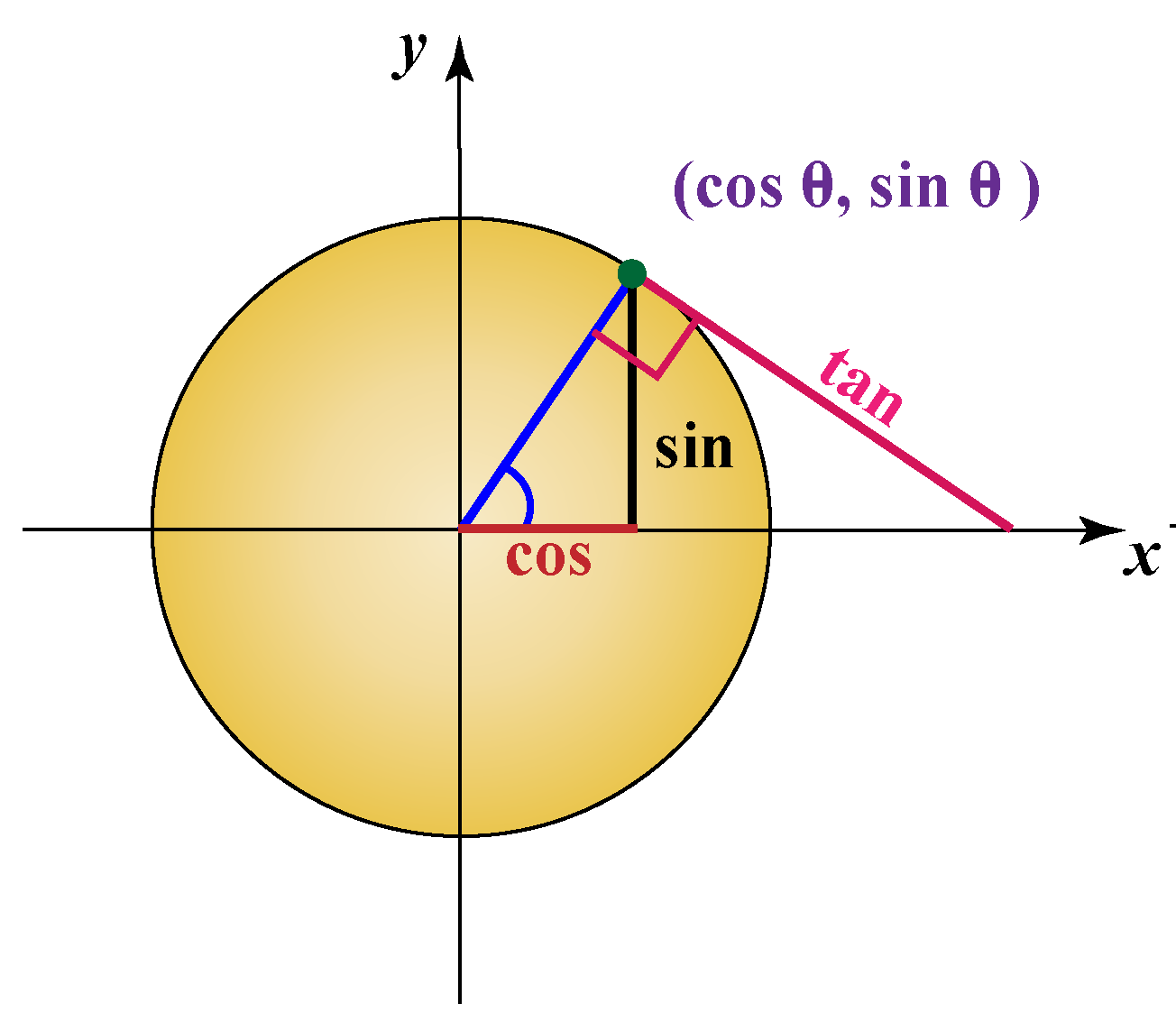Cosine is the x-coordinate and Sine is the y-coordinate.

Example when $$\theta = 0$$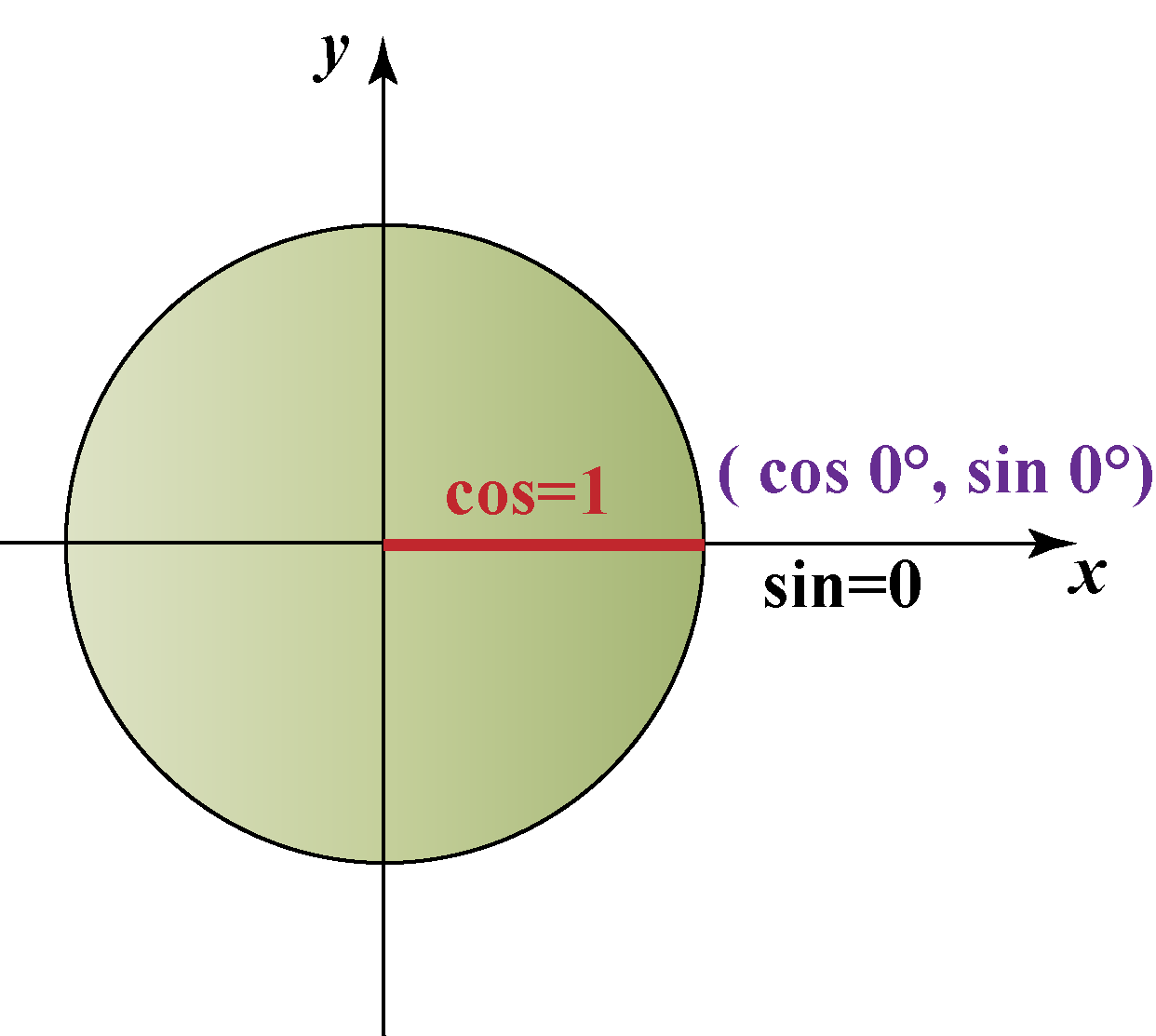x-coordinate is 1 and y-coordinate is 0

Therefore,

• $$\cos0^\circ = 1$$
• $$\sin 0^\circ = 0$$

Can you identify the value of $$\cos 90^\circ$$ and $$\sin 90^\circ$$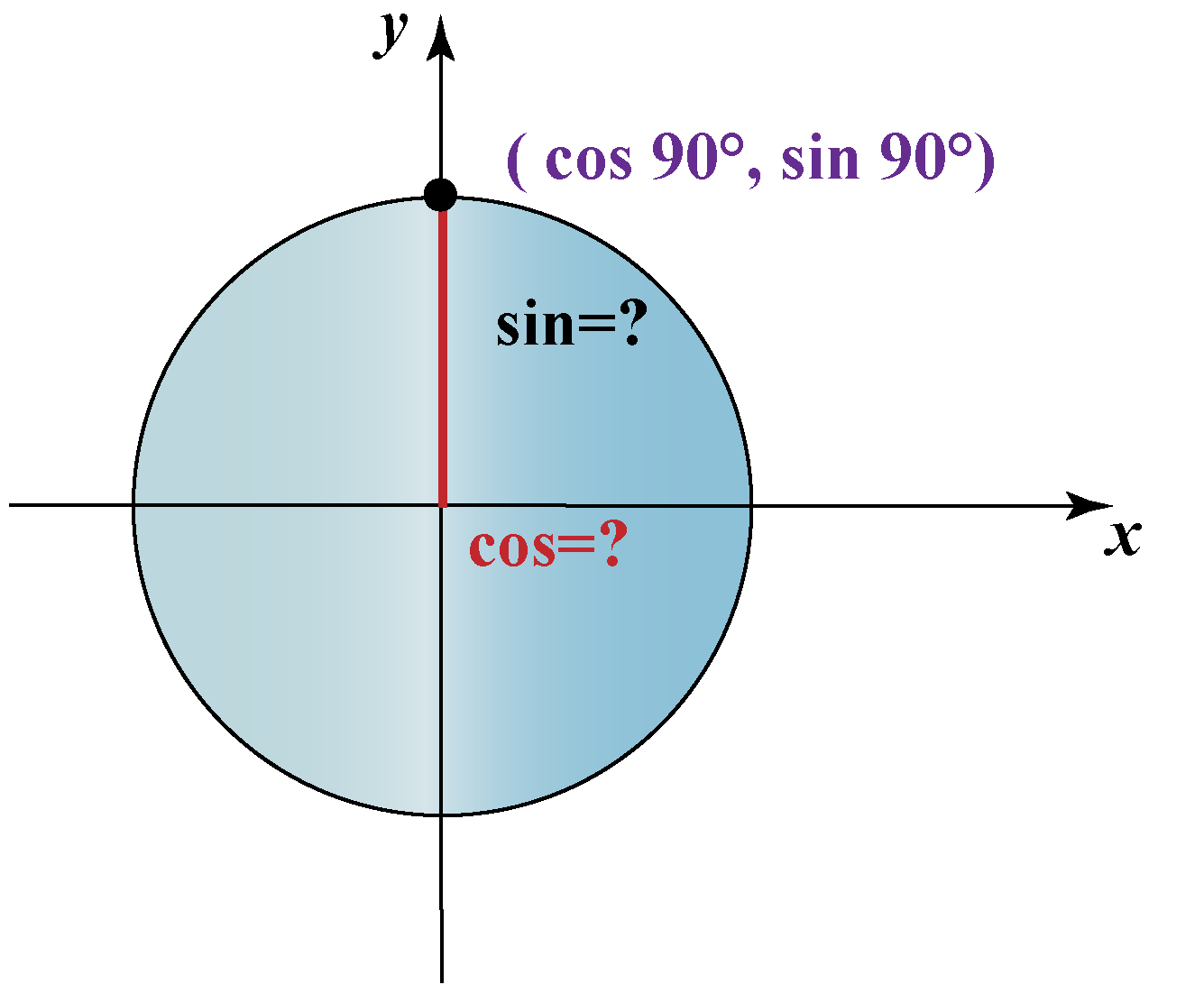Let us use this unit circle and find the important trigonometric function values when

$$\theta \text{ is}\: 30^\circ, 45^\circ, 60^\circ$$

Note that we can also measure in radians

We know that 360° = 2 $$\pi$$ Radians.

We can convert the angular measures to radian measures and express in terms of the radians.

Table- 1

Angle $$\theta$$ Radians Sin Cos Tan =\begin{align}\frac{\sin\theta}{\cos\theta} \end{align}
0° 0 0 1 0
30° \begin{align}\frac{\pi}{6}\end{align} \begin{align}\frac{1}{2}\end{align} \begin{align}\frac{\sqrt{3}}{2}\end{align} \begin{align}\frac{\sqrt{3}}{3}\end{align}
45° \begin{align}\frac{\pi}{4}\end{align} \begin{align}\frac{\sqrt{2}}{2}\end{align} \begin{align}\frac{\sqrt{2}}{2}\end{align} 1
60° \begin{align}\frac{\pi}{3}\end{align} \begin{align}\frac{\sqrt{3}}{2}\end{align} \begin{align}\frac{1}{2}\end{align} $$\sqrt{3}$$
90° \begin{align}\frac{\pi}{2}\end{align} 1 0 undefined

We can find the Secant, Cosecant and Cotangent functions using these formulas:

 Secant ($$\sec\theta$$ )= \begin{align}\frac{1}{\sin \theta}\end{align}
 Cosecant ($$\csc\theta$$ ) = \begin{align}\frac{1}{ \cos\theta}\end{align}
 Cotangent ($$\cot\theta$$ )= \begin{align}\frac{1}{\tan\theta}\end{align}

We have discussed the unit circle for the first quadrant.

Similarly, we can extend and find the radians for all the unit circle quadrants.

The numbers \begin{align} \frac{1}{2} , \frac{\sqrt 2}{2}, \frac{\sqrt3}{2}, 0\: \text{and}\:1 \end{align} repeat along with the sign in all 4 quadrants.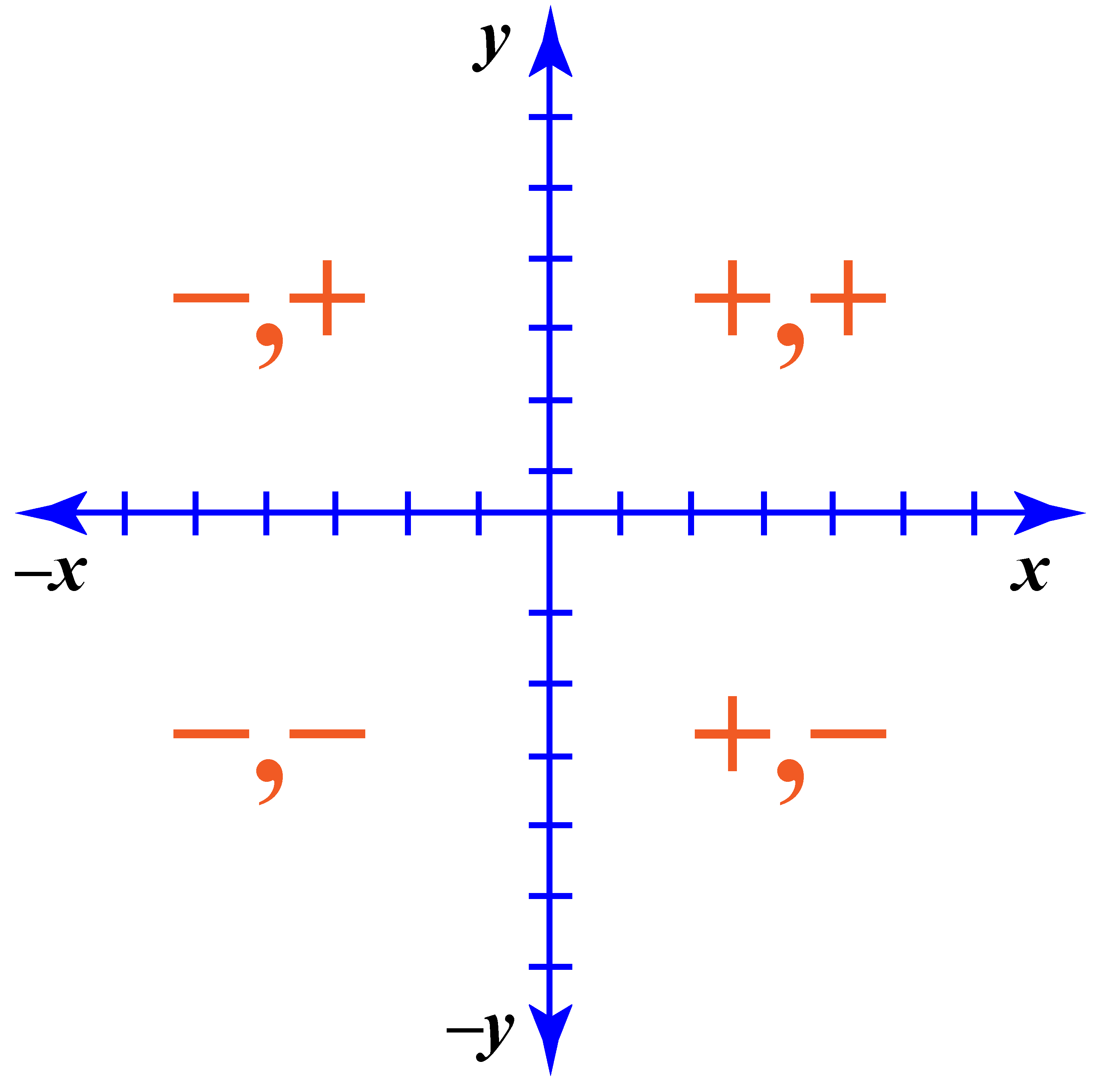Visit the unit circle simulation and verify the values at all four quadrants.

### Unit circle chart in Angular degrees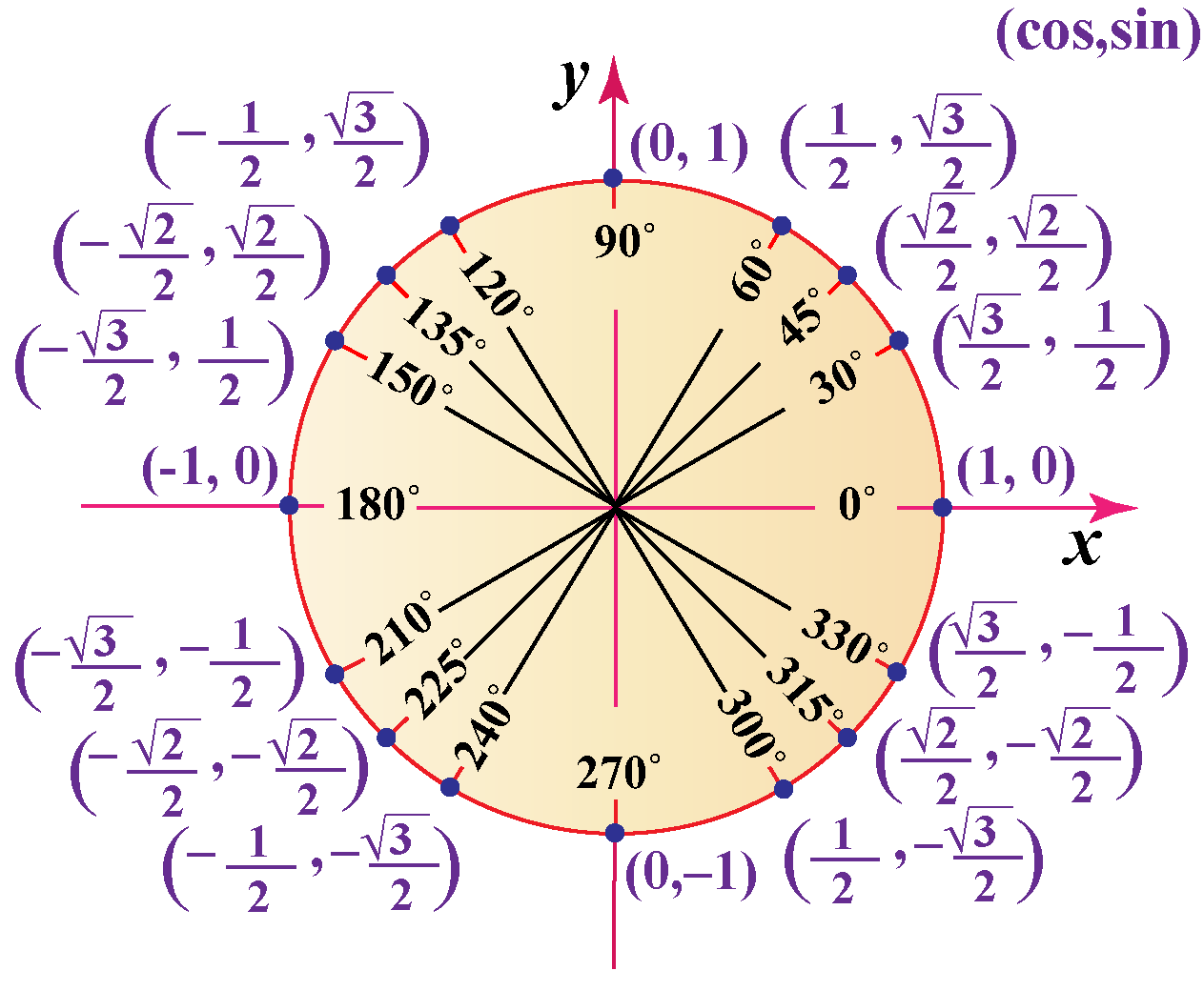### Unit circle chart in Radians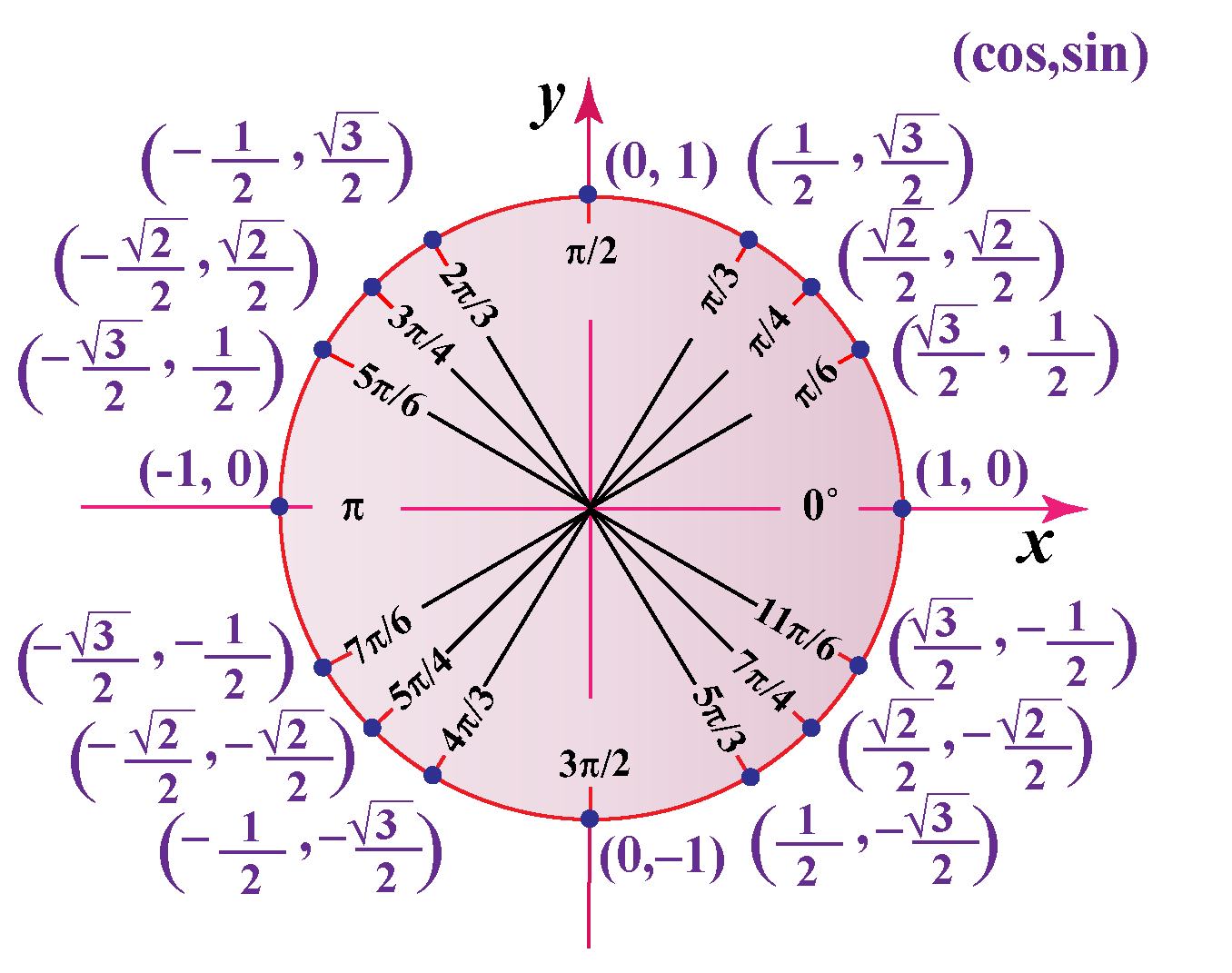### Unit circle Simulation

Select a Quadrant and drag the point P in the simulation below to visualise the unit circle in all four quadrants.Tips and Tricks
 1. All points on the unit circle alter between \begin{align}\frac{1}{2} , \frac{\sqrt 2}{2}, \frac{\sqrt3}{2}, \: 0 \:\text{and}\: 1 \end{align} (both positive and negative) Vertical lines indicate the sin value and horizontal lines indicate the cos value.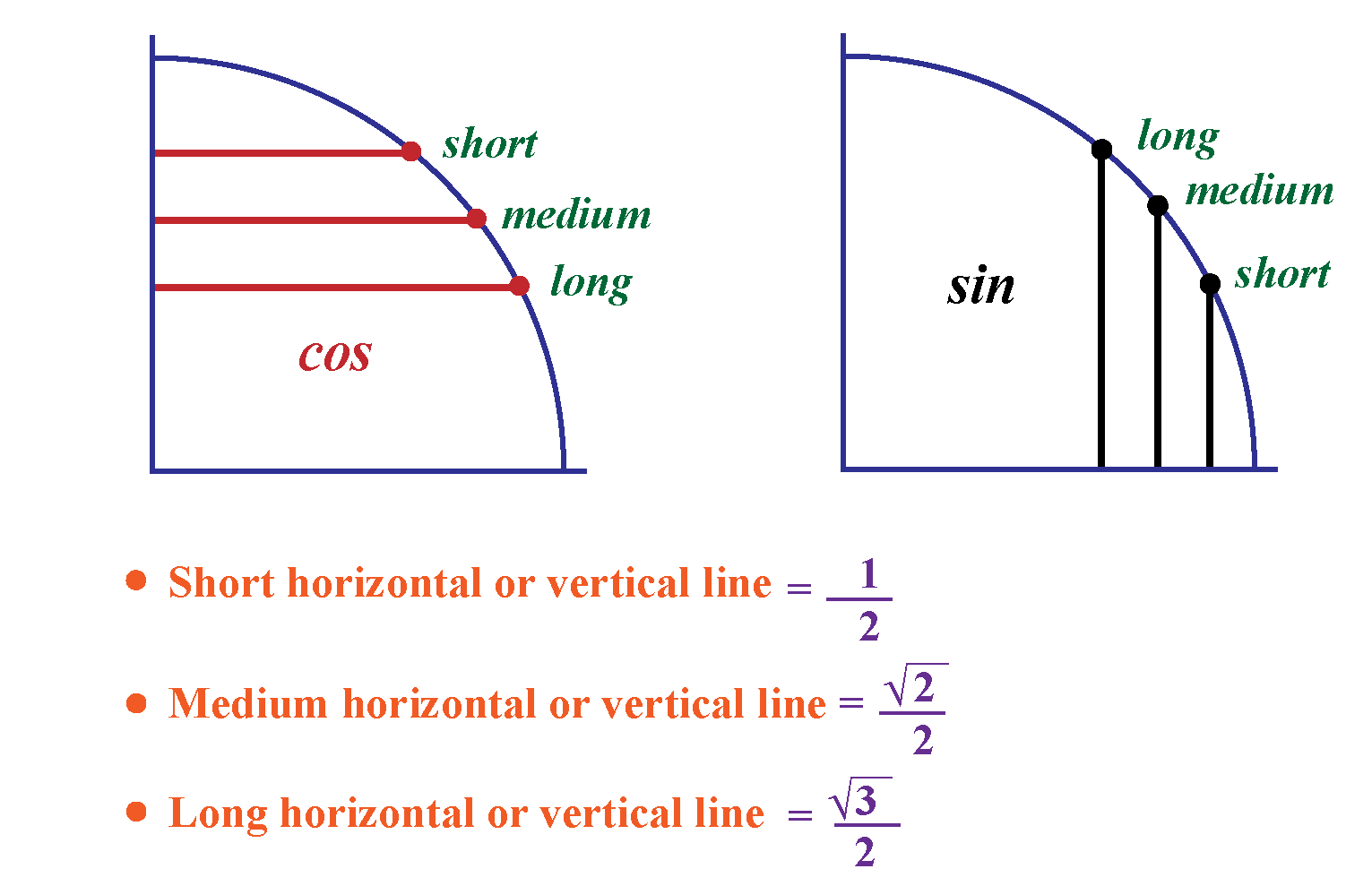## Unit Circle Calculator

Use the Unit circle calculator below to calculates the radians, sin, cosine and tan values.

Help your child score higher with Cuemath’s proprietary FREE Diagnostic Test. Get access to detailed reports, customised learning plans and a FREE counselling session. Attempt the test now.

## Solved Examples on Unit Circle Formula

 Example 1

Does the point P \begin{align}\left(\frac{1}{2}, \frac{1}{2} \right) \end{align} lie on the unit circle ?

Solution:

We know that equation of a unit cicle is

$$x^2 +y^2 = 1$$

Subsutituting \begin{align}x = \frac{1}{2}\end{align} and \begin{align}y = \frac{1}{2} \end{align}, we get:

\begin{align}&= x^2 +y^2 \\\\ &= \left(\frac{1}{2}^2 + \frac{1}{2}^2 \right) \\\\ &= \frac{1}{4} +\frac{1}{4} \\\\ &= \frac{1}{2}\\\\ &\neq 0 \end{align}

Since $$x^2 +y^2 \neq 1$$, the point P \begin{align}\left(\frac{1}{2}, \frac{1}{2} \right)\end{align} does not lie on the unit circle.

 $$\therefore$$ P \begin{align}\left(\frac{1}{2}, \frac{1}{2}\right)\end{align} ) doesn't lie on the unit circle.
 Example 2

Why is $$\cot 180$$° undefined?

Solution:

We know that

• \begin{align}\cot \theta = \frac{1}{\tan\theta}\end{align}
• \begin{align}\tan \theta = \dfrac{\sin \theta}{\cos\theta}\end{align}

Therefore

$$\cot \theta = \dfrac{\cos \theta}{\sin \theta}$$

From the unit circle chart, we know that

$$\sin 180^\circ = 0$$

Since division by 0 is $$\infty$$, $$\cot 180$$° = $$\infty$$

 $$\therefore \cot 180$$° = $$\infty$$
 Example 3

Use the unit circle chart and identify the following for the Point P\begin{align}\left(-\frac{\sqrt{2}}{2}, -\frac{\sqrt{2}}{2}\right) \end{align}

a) Angle $$\theta$$
c) sin $$\theta$$
d) cos $$\theta$$

Solution:Using the unit circle chart, we can say that:

P \begin{align}\left(-\frac{\sqrt{2}}{2}, -\frac{\sqrt{2}}{2}\right) \end{align} lies in the III quadrant.

The angle is 225°

• $$\sin$$ 225° is the y -coordinate \begin{align} -\frac{\sqrt{2}}{2}\end{align}
• $$\cos$$ 225° is the x-coordinate \begin{align}-\frac{\sqrt{2}}{2}\end{align}
 $$\therefore$$ The angle is  225° and it lies in the III quadrant.
 Example 4

Find the exact value of $$\tan$$ 210° using the unit circle.

Solution:

We know that

$$\tan 210°$$ = \begin{align}\frac{ \sin 210°}{ \cos210°}\end{align}

Using the unit circle chart:

• $$\sin$$ 210° =  -\begin{align}\frac{1}{2}\end{align}
• $$\cos$$ 210° = -\begin{align}\frac{\sqrt{3}}{2}\end{align}

Therefore,

\begin{align} \text{tan } 210° &= \frac{ \sin 210°}{\cos 210°} \\\\ &=\frac{-\frac{1}{2}}{-\frac{\sqrt{3}}{2}} \\\\ &=\frac{1}{\sqrt{3}} \\\\ &=\frac{\sqrt3}{3} \end{align}

 $$\therefore \tan$$ 210° = \begin{align}\frac{\sqrt{3}}{3}\end{align}
 Example 5

Find $$\sin$$ 900°

Solution:

The unit circle has  0°- 360°

Let us represent 900° in terms of 360°

900° is 2 full rotations of 360°  and an additional rotation of 180°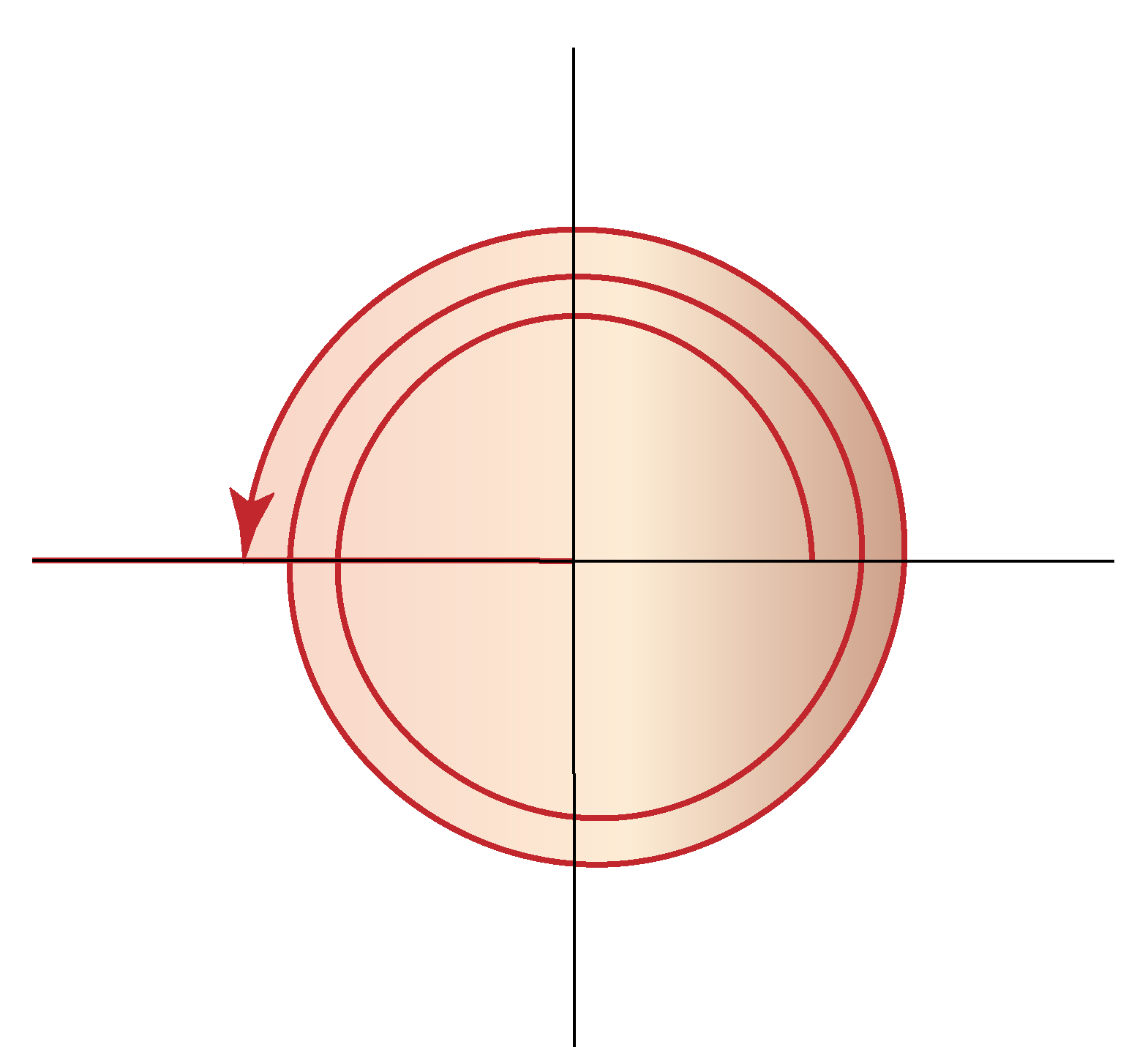Hence, 900° will have the same trigonometric ratio as 180°

Therefore,

$$\sin$$ 900° = $$\sin$$ 180°

From the unit circle chart, we know that:

$$\sin$$ 180° =  0

 $$\therefore \sin$$ 900° = 0

Have a doubt that you want to clear? Get it clarified with simple solutions on  Unit Circle from our Math Experts at Cuemath’s LIVE, Personalised and Interactive Online Classes.

Make your kid a Math Expert, Book a FREE trial class today!

## Practice Questions

Here are a few activities for you to practice. Select/Type your answer and click the "Check Answer" button to see the result.Challenging Questions
1.  Using the unit circle, prove the identity $$\sin^2 \theta +\cos^2 \theta = 1$$
2.  What are the sine, cosine and tangent for the x and y axes (recollect the angle formed at the axes)?

IMO (International Maths Olympiad) is a competitive exam in Mathematics conducted annually for school students. It encourages children to develop their math solving skills from a competition perspective.

## 1. What is a unit circle in math?

A unit circle is a circle of radius 1 unit.

## 2. What is the unit circle used for?

We use the unit circle to calculate the cosine, sine, and tangent of any angle between 0° and 360° ( 0 and 2π radians).

## 3. How does unit circle work?

A unit circle in the cartesian coordinate has (0,0) as it's center and radius 1 unit.

This creates a right triangle.

We can then apply the Pythagoras theorem to find the values of sin, cos and tan in the unit circle quadrants.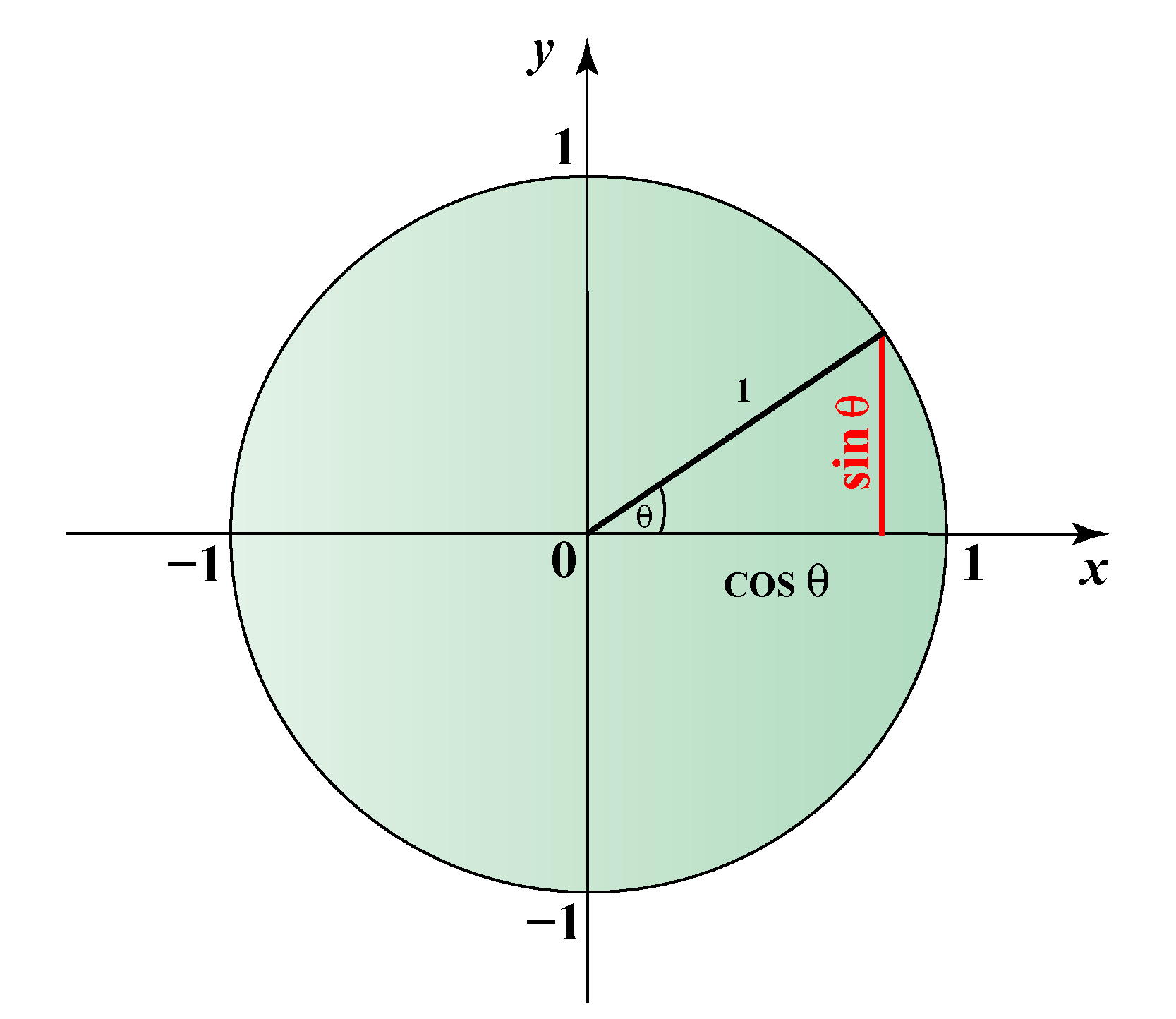## 4. How do you solve a unit circle problem?

Use the unit circle chart.

The point P gives the coordinates on the unit circle.

Use it to find the trigonometric function values.

For example: To find sin 225° and cos 225°, use the unit circle simulation above.

Fix θ as 225° and locate the coordinates.

x-coordinate gives the cosine and y-coordinate gives the sin values.

We can see that:

• sin 225° = -0.71
• cos 225° = -0.71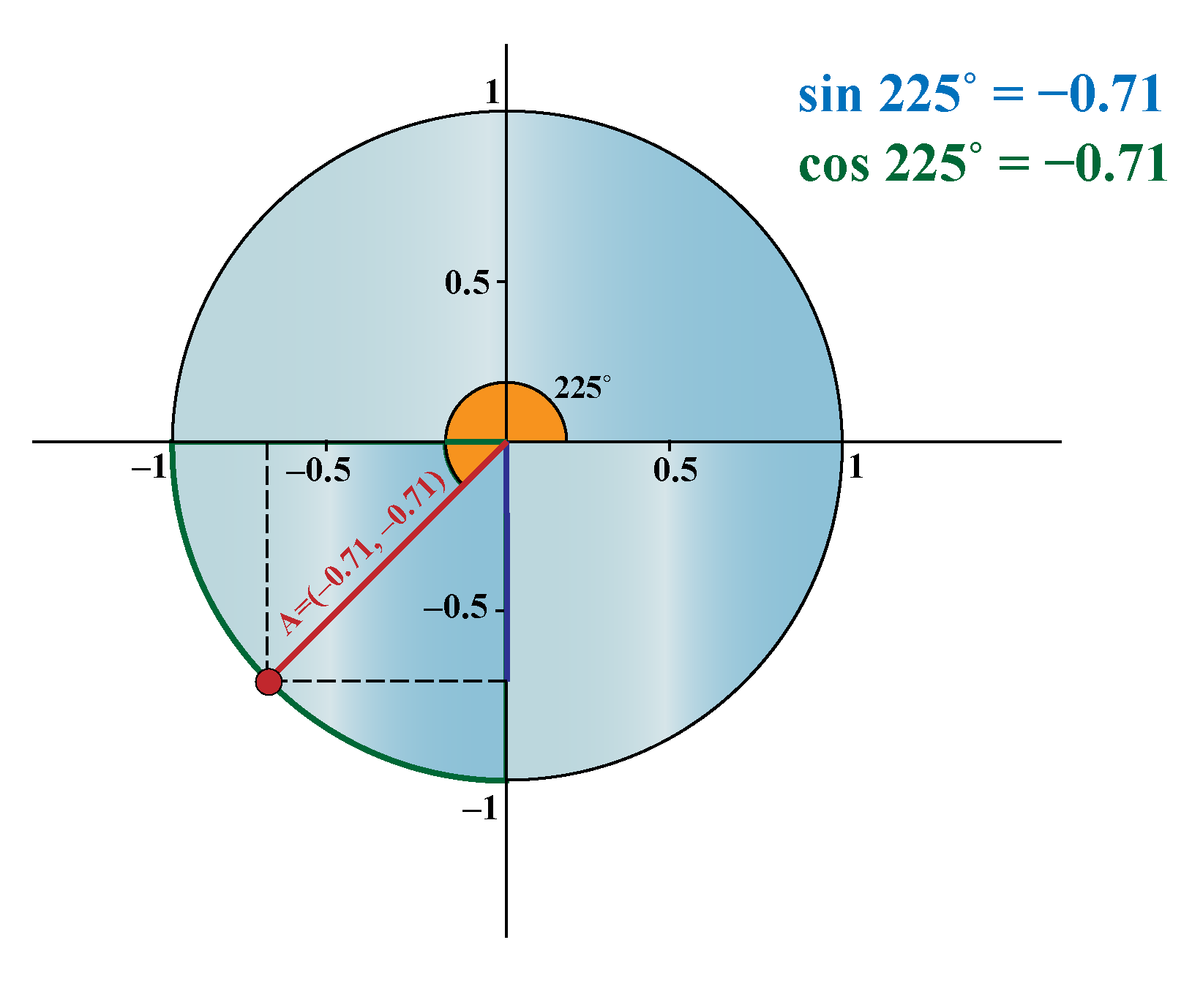More Important Topics
Numbers
Algebra
Geometry
Measurement
Money
Data
Trigonometry
Calculus